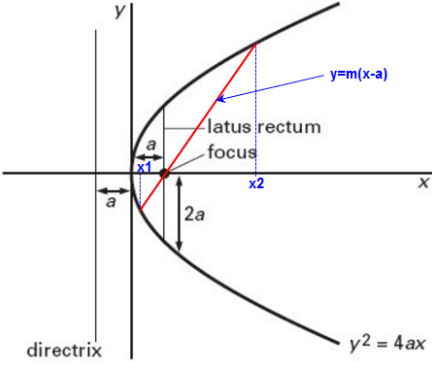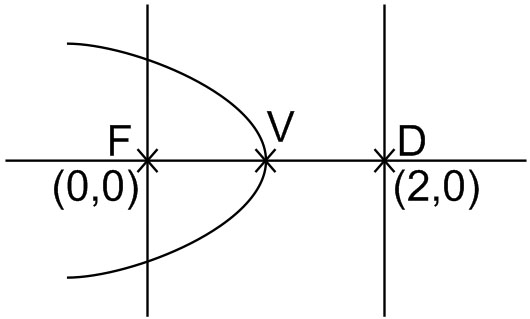Q

# How to solve this problem- - Co-ordinate geometry - JEE Main-4

A parabola has the origin as its focus and the line as the directrix. Then the vertex of the parabola is at

• Option 1)

(2, 0)

• Option 2)

(0, 2)

• Option 3)

(1, 0)

• Option 4)

(0, 1)

204 Views

As we learnt in

Standard equation of parabola -

- whereinVertex is mid-point of focus and foot of directrix.

V is (1,0)

Option 1)

(2, 0)

This option is incorrect.

Option 2)

(0, 2)

This option is incorrect.

Option 3)

(1, 0)

This option is correct.

Option 4)

(0, 1)

This option is incorrect.

Exams
Articles
Questions